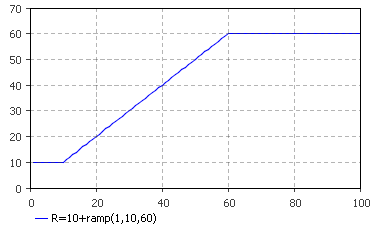AnyLogic

# ramp

ramp(double slope, double startTime, double endTime)

Returns 0 until the startTime and then slopes upward until endTime and then holds constant.

The plot below illustrates how the function works:#### Parameters

Name Type Description
slope double The coefficient of returned value growth between startTime and endTime.
startTime double The start time of the ramp.
endTime double The end time of the ramp.

#### Result

Type Description
double 0 until the startTime; then slopes upward until endTime and then holds constant.

#### Units

slope — units

startTime — time

pulseWidth — time

ramp() — units*time